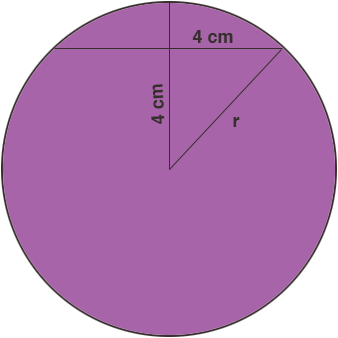SEARCH HOMEMath Central Quandaries & QueriesQuestion from girma, a student: one chord of a circle is 8cm long and it's distance from the center is 4cm long.what will be the length of another chord, of the same circle ,which is 2cm from the centerHi Girma,

Did you draw a diagram?Use Pythagoras' theorem to find the radius r.

REdraw the circle with a chord that is 2 cm from the center. This time you know the radius so you can use Pythagoras' theorem again to find half the length of the chord.

PennyMath Central is supported by the University of Regina and The Pacific Institute for the Mathematical Sciences.